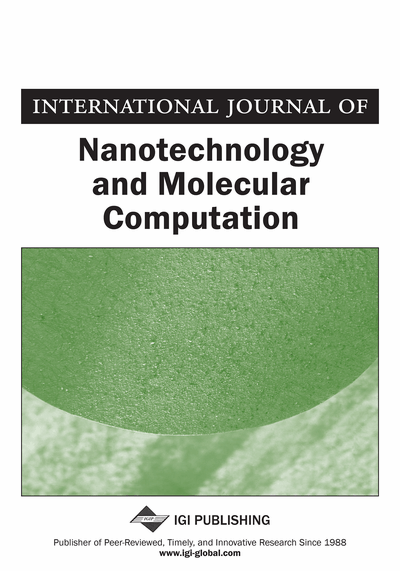# Computational Model Based on Evolutionary Primitives: Turing Machine Generalization

Oleg Granichin (Saint-Petersburg State University, Russia) and Valentin Vasilev (Saint-Petersburg State University, Russia)
DOI: 10.4018/jnmc.2010010103
Available
\$37.50
No Current Special Offers

## Abstract

This paper proposes generalization of Classical Turing machine scheme. New concept generalizes traditional concepts of â€œtapeâ€ and â€œtape cellâ€. In particular, â€œtape cellâ€ represents permanently running model of a dynamical system. â€œNaturalâ€ evolutions of cells proceed with jumpings. This model, for example, can describe systems with variable state spaces. Using this model one can avoid the problem of simulation continuous dynamic in the discrete time environment. Classical Turing machine is an extreme case of the proposed model.
Article Preview
Top

## Introduction

Turing machine (hereinafter, MT) is an abstract computing device, proposed in 1937 by mathematician Alan Turing (1936) as a mathematical model to describe algorithms. Initially, the concept of MT was being developed to answer the question whether for any mathematical statements it is possible to indicate the final sequence of instructions that could be carried out mechanically, one after another. In the same year A. Church (1936) proposed that any process which intuitively might be called a procedure could be implemented by a Turing machine. This hypothesis became known as the Church-Turing thesis.

Physical formulation of the Church-Turing principle: every finitely realizable physical system could be precisely modeled using the universal model of computer, operating by finite means. Practically, this set of finite resources is usually used as that of binary symbols (0, 1), and the fiinite realizability equivals to a finite number of cycles (the moments of movement of the head of the automaton).

However, physicists have emphasized the importance of quantum mechanics to computer science and the “exponential” advantage of quantum computers over classical. R. Feynman (1982) proved the impossibility of presenting the results of quantum mechanics in the classical universal computing device, even with the use of probabilistic algorithms. This is due to the fact that the number of elementary operations of the computing device will grow exponentially with the number of degrees of freedom. Therefore, for accurate simulation of quantum systems Feynman proposed a theoretical model of a quantum computer.

D. Deutsch (1985) proposed to modify the Church-Turing thesis as follows: “Each course realizable physical system can be fully simulated modeling universal computing machine operating by finite means.” D. Deutsch built a model of such a universal computer, based on the idea of quantum computing, and showed how a quantum computer can simulate physical systems, which are outside the area, affordable universal MT. Deutsch’s model gives a new perspective on the problem of modern computing devices, but in essence as well as the MT remains discrete.

To date uses of this theoretical results prognoses the miniaturization of all the practically working computational devices. Once assumed that more powerful machines will require more space for peripherals, memory, etc. This assumption was wrong. H. Moore (1965) formulated rule (later called Moore's Law), under which the performance of computer systems is doubling every eighteen months. H. Moore led his empirical law by constructing the dependence of the number of transistors in integrated circuit from time to time. As a consequence of this law we can derive the rate of miniaturization of the individual transistor. The development of digital electronic technology leads to the fact that the size of an elementary computing device is approaching the size of molecules or even atoms.

At this level begin to operate the quantum laws, which for many important dynamic problems have not been described theoretically. Increase the speed of computing devices and reduce their size inevitably leads to the necessity of operations with “transitional” processes. Instead of primitive operations with classical bits, it would be natural in the future to turn to the operations specified by those or other dynamic models of the microworld. Under the ‘’primitive’’ model of computation, one can, in particular, understanding and execution of operations with classical bits.

Of course, the introduction of a broader class of primitive models would be more justified if it were possible for a function whose values are recorded in the quantum register to define the operation, and implement effectively, for example, Fourier transform. And it may be a real suggestion that the time for its implementation will be quite comparable to the time of execution of one of the classical operations, as well as operations such as ‘’convolution’’ functions could easily be found in the ‘’nature’’. Recent studies of similar models show that their performance at the expense of the inherent nature of the capacity for self-organization is not necessarily ‘’expanded’’ into simpler components, i.e. not always be written in the form of the classical algorithm.

## Complete Article List

Search this Journal:
Reset
Volume 3: 4 Issues (2011)
Volume 2: 4 Issues (2010)
Volume 1: 4 Issues (2009)
View Complete Journal Contents Listing